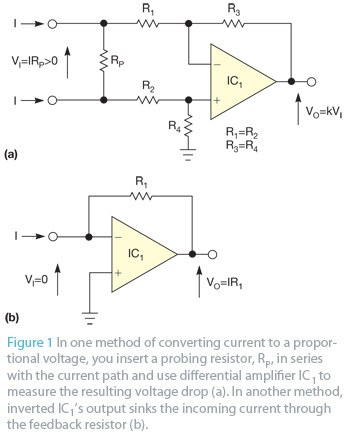# Measure small currents without adding resistive insertion lossIn most cases, you measure current by converting it into a proportional voltage and then measuring the voltage. Figure 1 shows two typical methods of making the conversion. In one method, you insert a probing resistor, RP, in series with the current path and use differential amplifier IC1 to measure the resulting voltage drop (Figure 1a). A second method is a widely known operational amplifier current-to-voltage converter in which inverted IC1’s output sinks the incoming current through the feedback resistor (Figure 1b).

In the first method, the same current that flows into one node flows from the second node, but a significant voltage drop occurs across probing resistor RP. In the second method, the voltage drop is on the order of tens of microvolts to millivolts, depending on IC1’s quality, but the measured current flows only into the sensing node with no return to the circuit. You can measure only currents flowing to ground.

The circuit in Figure 2 operates in a somewhat similar manner to the one in Figure 1b in that an op amp’s output sinks the incoming measured current. However, the other op amp’s output sources an equal outgoing current back to the circuit under test.

In Figure 2, input current I flows through R1 into the output of IC2, which reduces its voltage by the amount of IR1 relative to the input node. That voltage equals the voltage mean of the op amp’s outputs, which R3 and R4 set at the op amp’s inverting inputs. Consequently, the output of IC1 must rise to a voltage of IR2 relative to the inverting inputs and the equal-voltage noninverting input node of IC2. IC1 sources this current, which returns through R2 to the circuit under test. R1=R2, so the output current is the same as the input current. Because the op amp’s outputs maintain their inputs at equal voltages, the circuit under test has virtually no resistance.

READ  Inside the vintage 74181 ALU chip: how it works and why it's so strange